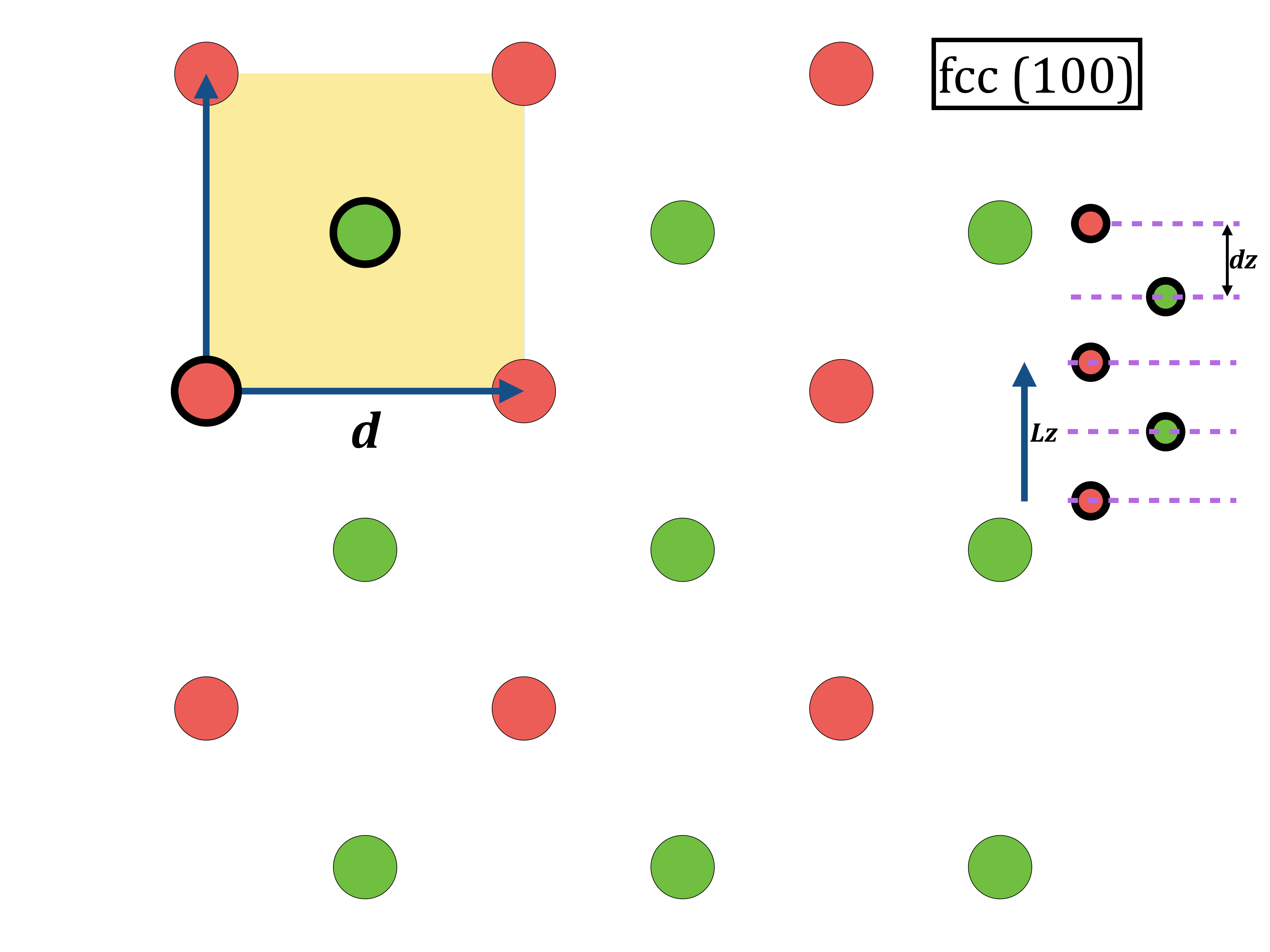#CP2K Open Source Molecular Dynamics

### Site Tools

exercises:2015_ethz_mmm:surface_cu

# Surface energies of Copper high-symmetry surfaces

TO USE THE FUNCTION LIBRARY (VERSION UP TO DATE) IN THE INTERACTIVE SHELL:

you@eulerX ~$module load courses mmm vmd you@eulerX ~$ mmm-init

REMEMBER: this is the command to load the module for the cp2k program:
you@eulerX ~$module load new cp2k and to submit the job: you@eulerX ~$ bsub < jobnameIn this exercise we will compute the surface energies of Cu using the EAM potential. As a reference, we report the table from the Gross book:

• Download all the necessary files from from the wiki: exercise_3.1.zip (all inputs are commented) in your home directory and unzip it:
you@eulerX ~$wget http://www.cp2k.org/_media/exercises:2015_ethz_mmm:exercise_3.1.zip you@eulerX ~$ unzip exercises:2015_ethz_mmm:exercise_3.1.zip
you@eulerX ~$cd exercise_3.1 • Run the optimizations 100.inp, 110.inp, 111.inp and the bulk. you@eulerX exercise_3.1$ bsub cp2k.popt -i 100.inp -o 100.out
you@eulerX exercise_3.1$bsub cp2k.popt -i 110.inp -o 110.out you@eulerX exercise_3.1$ bsub cp2k.popt -i 111.inp -o 111.out
you@eulerX exercise_3.1$bsub cp2k.popt -i bulk.inp -o bulk.out • While geometry optimization is running you can have a look at the corresponding initial coordinate files 100.xyz, 110.xyz, 111.xyz. In vmd it is also possible to open a console, and give the command pbc set { a b c 90 90 90 } where a, b, c can be extracted from the cp2k input file. Then you can make several periodic copies for visualization. you@eulerX exercise_3.1$ vmd 100.xyz
you@eulerX exercise_3.1$vmd 110.xyz you@eulerX exercise_3.1$ vmd 111.xyz
• Compute the three surface energies: you need to compute the area, subtract bulk contribution, take care of the units.
• Unordered List ItemIn the class we will learn how to compute the Wulff crystal from these three numbers.
• Basically, you should edit the input file “DP”, replacing “PUT_HERE_THE_XXX_SURFACE_ENERGY” with corresponding surface energy. Note that for the {100} and {110} surfaces you need to edit TWO lines each: the program takes care of assigning the surface energy to equivalent surfaces (like (010)) but since it was developed for island on surfaces it does not do it automatically in all directions. z direction is treated differently in this program.
• At this point, you can run the sowos program:
you@eulerX exercise_3.1$./sowos.v02.00.02.x • There will be many output files. Important are: 1. the atomistic model out.atomistic-inside-gnuplot.xyz which will contain many atoms if you chose a proportionality constant in the file DP which is too large. If you appropriately modify it (how?) you can have a xyz file to be opened in vmd. 2. the out.plot-gnuplot.plt file. You can open it with gnuplot: you@eulerX exercise_3.1$ gnuplot
gnuplot> replot